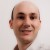# how to calculate ppm?

Six Sigma – iSixSigma Forums Old Forums General how to calculate ppm?

Viewing 6 posts - 1 through 6 (of 6 total)
• Author
Posts
• #36372

Lot Size  Die size(mm)  Sample size No. of non-conforming units

1700k      0.6*0.6           4200                          10

500k        0.7*0.7           1400                            3

100k          0.6                  500                            0

Calculate the total ppm with all lot sizes :
ppm=10/4200*1million+3/1400*1million+0
= 4523.8  » 4524ppm
Calculate ppm with the first and second lot sizes:
ppm=10/4200*1million+3/1400*1million
= 4524 ppm
Calculate ppm with the first lot size:
ppm= 10/4200*1 million
=2381ppm
Calculate ppm with the second lot size:
ppm= 3/1400*1million
=2143ppm
I have calculated the above answers, but my boss told me that I did not consider the lot size during calculating ppm. Can anyone tell what’s wrong with me?

Thanks,

Chris

0
#104985

Whats your problem dude?
DD

0
#104998

My boss told me that I calculated wrong. He said that I haven’t considered the population. Do you think that my calculation are wrong?
Thanks,
Chris

0
#105005Gabriel
Participant

There are a couple of problems here.
To begin with, your boss is right. You are not taking into account the population sizes. Further more, with your reasoning if you had 3 lots of 400,000PPM each the total PPM would be 1.2 millions fefective per million parts! (you cannot have more defective parts than parts)
For example, lot 1:
lot size: 1700k units
sample size: 4200 units
defective units found in the sample: 10
PPM of the sample: 10/4200*1M=2381PPM (the same figure you got)
If you take that value as the estimation of the PPM in the lot, then you can estimate 1700k*2381/1M=4048 as the number of defectives in the lot.
Making the same reasoning for the second lot, you can estimate the number of defectives to be 500k*2143/1M=1071.
Then, the number of defectives in lots 1+2 is 4048+1071=5199. As the total number of units in lots 1+2 is 1700k+500k=2200k=2.2M, the PPM for lots 1+2 is 5199/2.2M*1M=2363 PPM.
Another problem is the reliability of the sample PPM as an estimate of the population PPM. With so few defectives found in the sample, the uncertainty due to sampling variation is high. For example, these are the 90% confidence intervals (i.e. the range where you expect with a 90% of confidence that the ture lot PPM will be) for the three lots based what was found in the sample:
Lot 1: 1469<PPM<4035
Lot 2: 997<PPM<5528
Lot 3: PPM<5973
As you see, there is a wide overlapping, so it is even possible that all lots have the same PPM.
For more info about sampling variation and PPM, try this link to a previos post from today:
https://www.isixsigma.com/forum/showmessage.asp?messageID=51875

0
#105053qurious
Participant

Gabriel,
Please let me know how you arrived at below figures(calculation)
Lot 1: 1469<PPM<4035
Lot 2: 997<PPM<5528
Lot 3: PPM<5973
Regards

0
#105130Gabriel
Participant

Using the binomial distribution. The defects in a lot are binomially distributed IF the defects were produced at random OR the sample is taken at random (or both), as long as the sample is taken with repetition (a sampled part is returned to the lot after sampling, existing a probability to take the same part twice) OR the lot is infinitely large. In all these cases the lot is many times larger than the sample, so the “infinitely large lot” is a very good model.
Let’s call p the actual defectives rate in the lot (that you don’t know and will not know either), n the sample size, and X1 the number of defecives found in the sample. X will be binomially distributed. Let’s work with P(X<=X1), which is the probabilty that X1 or fewer defectives are found in a sample of size n taken form a lot with a fraction p defective.
I don’t remember the figures now, but let’s say that in a sample of 1000 I find 5 defectives. The point estimation for p is 5/1000=0.005, or if you prefer 0.5% or 5000PPM. However, it is perfectly understandable that the lot does not NEED to have 0.5% defective. But it is also clear that it is very unlikely that find “only” 5 bad out of 1000 if 30% was bad in the lot, or that you find as much as 5 out of 1000 if the lot had only 4.3PPM. This intuitive feeling can be shown with the probability:
We have n=1000, X1=5.
Let p=0.3 (30%) ==> P(X<=X1)=P(X<=5)=1.5×10^(-144), that's zero poin 143 zeros befor the first non zereo decimal. Do you let me say impossible?
Let p=0.0000043 (4.3PPM) ==> P(X<=X1)=P(X5 is impossible.
Clearly the result of these probabilities are too extremme.
I said the figures were the 90% confidence interval. The 90% confidnece interval that that leaves 5% of chances on each side. That means that we must find:
p such as P(X<=5)=0.05, and
p such as P(X<=5)=0.95
These p have to be found by trial and error (or an iterative method). Computers can certainly make your live easier here.
In this example, I found p=0.01 (1%) and p=0.0026 (0.26%). This is the 90% confidence interval. Compare with the point estimation of 0.5%.
It is interesting to note the effect of the sample size. If you find 50 in 10,000, the point estimation remains 0.005 (5%), but the 90% confidence interval narrows to 0.4% ~ 0.6% (compare with 0.26% ~ 1.0%).
Now, if your question what is the formula, then the formula you need is the one to calculate the cummulative probability in a binomial distribution. Go to any statistics book or website or use the BINOMDIST function in Excel.

0
Viewing 6 posts - 1 through 6 (of 6 total)

The forum ‘General’ is closed to new topics and replies.